# Dichotomy method

(diff) ← Older revision | Latest revision (diff) | Newer revision → (diff)

method of division in halves

A method for numerically solving equations in a single unknown. Consider the equation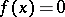with a continuous functionon the intervalwhich takes values of different signs at the end points of the interval and which has a single root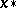within. To findapproximately, one dividesinto halves and calculates the value of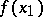at the midpoint. If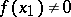, one takes the two intervals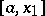and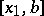and from them selects for the next dichotomy the one at the end points of which the values of the function differ in sign. This continued division into halves gives a sequence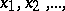which converges to the rootwith the rate of a geometrical progression: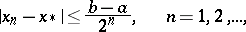(1)

where the bound (1) cannot be improved upon in this class of functions. Ifhas more than one root in, the sequence will converge to one of them.

A method for minimizing a function of one variable. One has to find the minimumof a unimodal functionon an intervaland to determine the pointat which it is attained. For this, one dividesinto halves and near the middle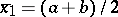calculates the values ofat the two points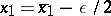and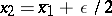, where the numberis a parameter of the method and is sufficiently small. Then the valuesand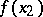are compared, and on the basis thatis unimodal one selects from the two intervalsandthe one that certainly contains. For example, if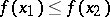, this will be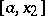, otherwise. The interval is again divided into halves, and near the middleone takes two points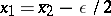and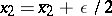, compares the values of the function, etc. As a result, one obtains a sequence of midpoints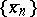for which(2)

As an approximation to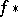the value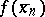for sufficiently largeis taken.

The name is given to the method because at each step in this algorithm the segment containing the minimum becomes approximately half the length. The dichotomy method is not the best in the class of unimodal functions. There are more effective methods that enable one to use the same number of calculations on the values of the function to obtain an accuracy better than that of (2) (see, for example, the Fibonacci method).

How to Cite This Entry:
Dichotomy method. Encyclopedia of Mathematics. URL: http://encyclopediaofmath.org/index.php?title=Dichotomy_method&oldid=12591
This article was adapted from an original article by M.M. Potapov (originator), which appeared in Encyclopedia of Mathematics - ISBN 1402006098. See original article